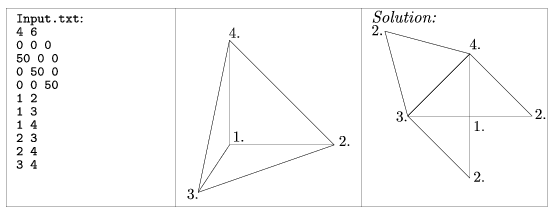Mathematical and Physical Journal
for High Schools
Issued by the MATFUND Foundation
 Already signed up? New to KöMaL?

#Problem S. 22. (December 2006)

S. 22. In this exercise your program should draw the planar net of a given convex polyhedron.

A convex polyhedron is a 3 dimensional solid bounded by planes and its vertices and edges will be given. The net of the polyhedron should be cut at appropriate points and drawn in the plane without distortion. It is required that polygons in the planar net and corresponding faces of the polyhedron be congruent. The planar net should not overlap itself and should be connected.

The name of the input file is given as a command line argument as s22.exe Input.txt.

The first line of the input file contains two integers 3<n<30 and 6<k<300. Then 3 integers separated by spaces stand in each of the following n lines, representing the (x,y,z) coordinates of a vertex. The next k lines contain 2 integers each, representing the numbers of vertices on the same edge of the polyhedron. The smallest vertex number is 1, referring to the vertex in the second line of the input file.

Example:The source code of the program (s22.pas, s22.cpp, ...) with some remarks and a short documentation (s22.txt, s22.pdf, ...) should be submitted.

(10 pont)

Deadline expired on January 15, 2007.

Sorry, the solution is available only in Hungarian. Google translation

Az alábbi file tartalmazza a mintamegoldást, illetve a javításhoz használt tesztadatokat.

### Statistics:

 5 students sent a solution. 10 points: Gilián Zoltán. 8 points: 1 student. 7 points: 1 student. 5 points: 1 student. 0 point: 1 student.

Problems in Information Technology of KöMaL, December 2006